# Variables

## What is an experimental variable in OpenSesame?

Experimental variables in OpenSesame are those variables that:

• You can refer to in the user interface with the '{variable_name}' syntax.
• Are available as global variables in a Python inline_script.
• Are available as global variables in a JavaScript inline_javascript.
• Contain things like:
• The variables that you have defined in a loop item.
• The responses that you have collected.
• Various properties of the experiment.
• Etc.

## The variable inspector

The variable inspector (`Ctrl+I`) provides an overview of available variables (Figure 1). When the experiment is not running, this overview is based on a best guess of which variables will become available during the experiment. However, when the experiment is running, the variable inspector shows a live overview of variables and their values. This is useful for debugging your experiment.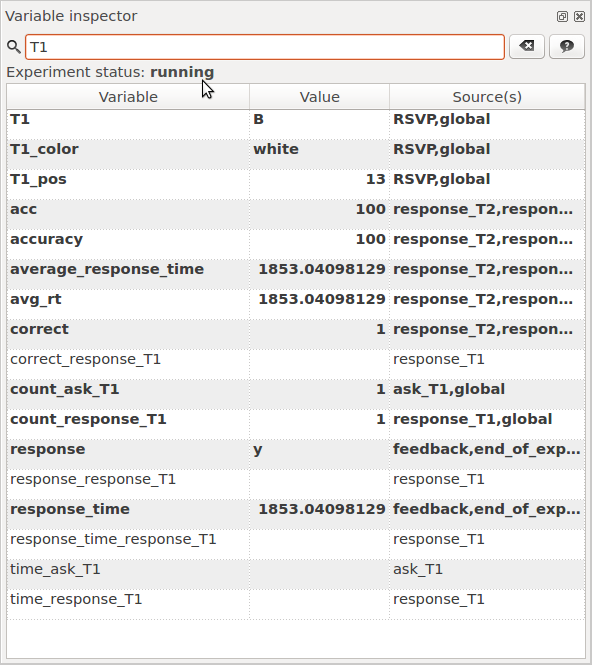Figure 1. The variable inspector provides an overview of all variables that OpenSesame knows about.

## Defining variables

The simplest way to define a variable is through the loop item. For example, Figure 2 shows how to define a variable named `gaze_cue`. In this example, trial_sequence item is called four times while `gaze_cue` is 'left' and another four times while 'gaze_cue' is 'right'.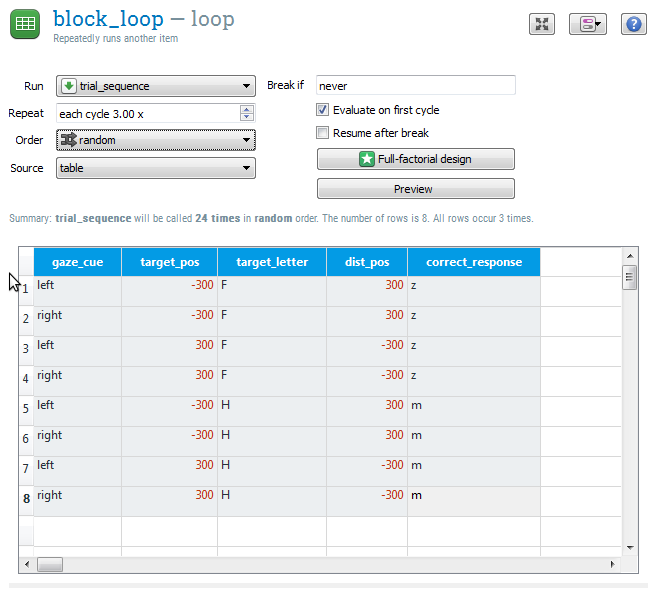Figure 2. The most common way to define independent variables is using the loop table.

## Built-in variables

The following variables are always available:

### Experiment variables

Variable name Description
`title` The title of the experiment
`description` The description of the experiment
`foreground` The default foreground color. E.g., 'white' or '#FFFFFF'.
`background` The default background color. E.g., 'black' or '#000000'.
`height` The height-part of the display resolution. E.g., '768'
`width` The width-part of the display resolution. E.g., '1024'
`subject_nr` The subject number, which is asked when the experiment is started.
`subject_parity` Is 'odd' if `subject_nr` is odd and 'even' if `subject_nr` is even. Useful for counterbalancing.
`experiment_path` The folder of the current experiment, without the experiment filename itself. If the experiment is unsaved, it has the value `None`.
`pool_folder` The folder where the contents of the file pool have been extracted to. This is generally a temporary folder.
`logfile` The path to the logfile.

### Item variables

There are also variables that keep track of all the items in the experiment.

Variable name Description
`time_[item_name]` Contains a timestamp of when the item was last executed. For sketchpad items, this can be used to verify the timing of display presentation.
`count_[item_name]` Is equal the number of times minus one (starting at 0, in other words) that an item has been called. This can, for example, be used as a trial or block counter.

### Response variables

When you use the standard response items, such as the keyboard_response and mouse_response, a number of variables are set based on the participant's response.

Variable name Description
`response` Contains the last response that has been given.
`response_[item_name]` Contains the last response for a specific response item. This is useful in case there are multiple response items.
`response_time` Contains the interval in milliseconds between the start of the response interval and the last response.
`response_time_[item_name]` Contains the response time for a specific response item.
`correct` Is set to '1' if the last `response` matches the variable `correct_response`, '0' if not, and 'undefined' if the variable `correct_response` has not been set.
`correct_[item_name]` As `correct` but for a specifc response item.

### Feedback variables

Feedback variables maintain a running average of accuracy and response times.

Variable name Description
`average_response_time` The average response time. This is variable is useful for presenting feedback to the participant.
`avg_rt` Synonym for `average_response_time`
`accuracy` The average percentage of correct responses. This is variable is useful for presenting feedback to the participant.
`acc` Synonym for `accuracy`

## Using variables in the user interface

Wherever you see a value in the user interface, you can replace that value by a variable using the '{variable name}' notation. For example, if you have defined a variable `soa` in a loop item, you can use this variable for the duration of a sketchpad as shown in Figure 3.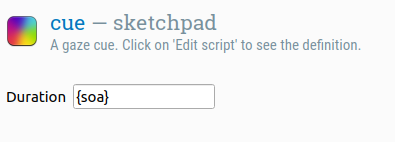Figure 3. The duration '{soa}' indicates that the duration of the sketchpad depends on the variable `soa`.

This works throughout the user interface. For example, if you have the defined a variable `my_freq`, you can use this variable as the frequency in a synth item, as shown in Figure 4.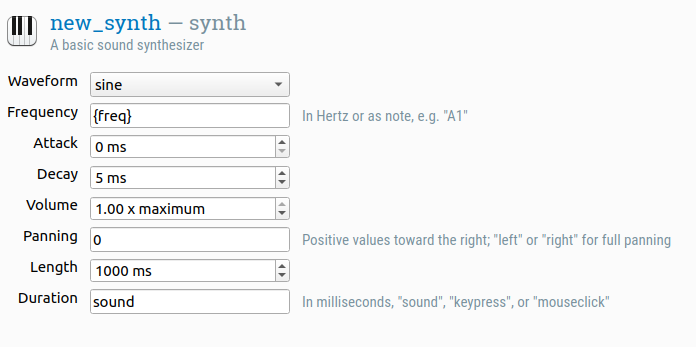Figure 4. The frequency '{my_freq}' indicates that the frequency of the synth depends on the variable `my_freq`.

Sometimes, the user interface doesn't let you type in arbitrary text. For example, the elements of a sketchpad are shown visually, and you cannot directly change an X coordinate to a variable. However, you can click on the Select view → View script button on the top right, and edit the script directly.

For example, you can change the position of a fixation dot from the center:

``````draw fixdot x=0 y=0
``````

… to a position defined by the variables `xpos` and `ypos`:

``````draw fixdot x={xpos} y={ypos}
``````

## Using Python expressions in the user interface

When referring to variables using the `{my_var}` notation, you are in fact using a so-called f-string, which is a way to embed Python code in strings of text. You can also use f-strings to evaluate arbitrary Python code. For example, you can multiply the variables `width` and `height` and include the result in a sketchpad, like so: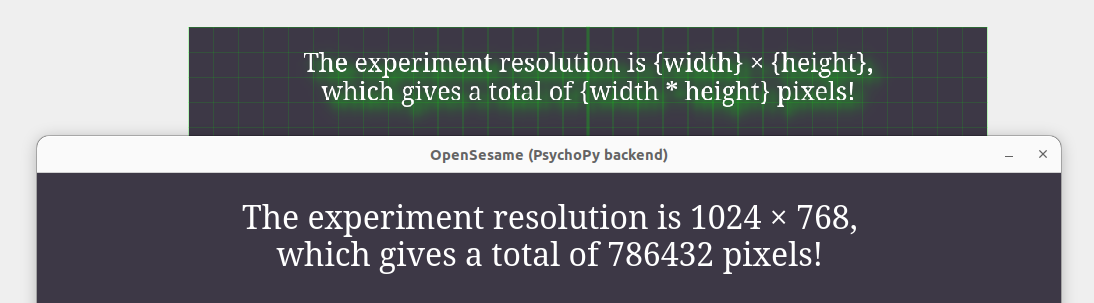Figure 5. You can embed Python expressions using f-strings.

f-strings are Python code, and are therefore only supported on the desktop, but see below for a JavaScript alternative for browser experiments.

## Using JavaScript expressions in the user interface

When using OSWeb, expressions included between curly braces are interpreted as template literals. This is very similar to f-strings in Python, with the important difference that it uses JavaScript.

In normal JavaScript, expressions inside template literals are prefixed with a `\$`, like so: `\${expression}`. This is allowed in OpenSesame but not necessary: the prefix is automatically added to improve compatibility between browser and desktop experiments. In most cases, as in the figure below, the exact same expression is valid as a Python f-string on the desktop and a JavaScript template literal in the browser.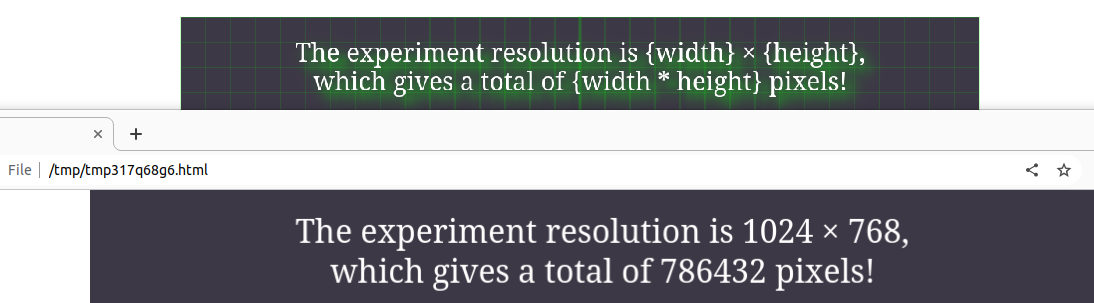Figure 6. You can embed JavaScript expressions using template literals.

## Using variables in Python

In an inline_script, experimental variables are available as global variables. For example, if you have defined `example_variable` in a loop, then the following will print the value `example_variable` to the debug window:

``````print(example_variable)
``````

You can set the experimental variable `example_variable` to the value 'some value' as follows:

``````example_variable = 'some value'
``````

## Using variables in JavaScript

In an inline_javascript, experimental variables are available as global variables. For example, if you have defined `example_variable` in a loop, then the following will print the value `example_variable` to the browser console:

``````console.log(example_variable)
``````

You can set the experimental variable `example_variable` to the value 'some value' as follows:

``````example_variable = 'some value'
``````

## Using conditional ("if") statements

Conditional statements, or 'if statements', provide a way to indicate that something should happen only under specific circumstances, such when some variable has a specific value. Conditional statements are regular Python expressions.

The most commonly used if-statement in OpenSesame is the run-if statement of the sequence, which allows you to specify the conditions under which a particular element is executed. If you open a sequence item, you see that every item from the sequence has a 'Run if …'' option. The default value is 'always', which means that the item is always run; but you can also enter a condition here. For example, if you want to show a green fixation dot after a correct response, and a red fixation dot after an incorrect response, you can create a sequence like the following (this makes use of the fact that a keyboard_response item automatically sets the `correct` variable, as discussed above) as shown in Figure 7.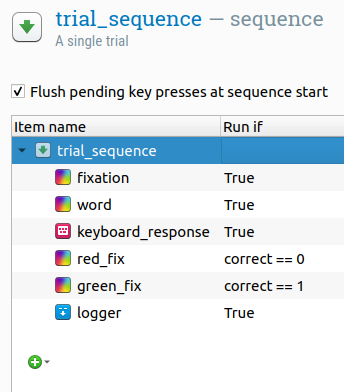Figure 7. 'Run if' statements can be used to indicate that certain items from a sequence should only be executed under specific circumstances.

You can use more complex conditions as well. Let's take a look at a few examples:

``````correct == 1 and response_time > 2000
correct != 1 or response_time > max_response_time or response_time < min_response_time
``````

The same principle applies to 'Show if' fields in sketchpad items. For example, if you want to draw a right-upwards-pointing arrow only if the variable `quadrant` has been set to 'upper right', simply type the proper condition in the 'Show if ...' field and draw the arrow, as in Figure 8. Make sure that you draw the arrow after you have set the condition.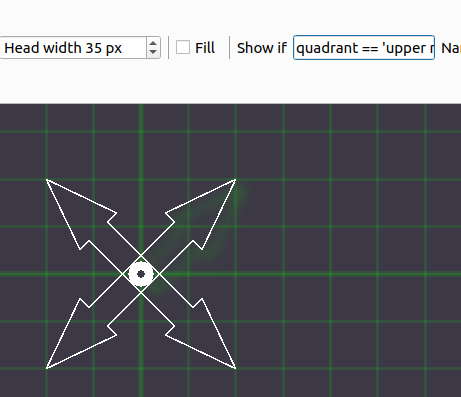Figure 8. 'Show if' statements can be used to indicate that certain elements from a sketchpad or feedback item should only be shown under specific circumstances.

Important: The moment at which a conditional statement is evaluated may affect how your experiment works. This is related to the prepare-run strategy employed by OpenSesame, which is explained here: